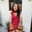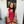Trusted answers to developer questions
Trusted Answers to Developer Questions

Related Tags

# How to break a single byte key XOR encryptionAqsa Amir

Grokking Modern System Design Interview for Engineers & Managers

Ace your System Design Interview and take your career to the next level. Learn to handle the design of applications like Netflix, Quora, Facebook, Uber, and many more in a 45-min interview. Learn the RESHADED framework for architecting web-scale applications by determining requirements, constraints, and assumptions before diving into a step-by-step design process.

### Overview

XOR encryption belongs to the category of symmetric encryption, where the same key is used to encrypt and decrypt the message.

The XOR encryption and decryption method

### Breaking XOR encryption

Single byte key XOR encryption is easier to break because the key is only 8 bits long. The following steps define a method of breaking XOR encryption:

### Step 1

Convert the cipher text into binary and make groups of 8 bits each.

Since the example cipher text is in hexadecimal, we will convert each character into bits of length four and concatenate two characters to generate one byte each.

#include <iostream>#include <bits/stdc++.h>using namespace std;//function to convert hexadecimal string to binarystring HexToBin(string hexdec){    long int i = 0;     string binary = "";    while (hexdec[i]) {         switch (hexdec[i]) {        case '0':            binary += "0000";            break;        case '1':            binary += "0001";            break;        case '2':            binary += "0010";            break;        case '3':            binary += "0011";            break;        case '4':            binary += "0100";            break;        case '5':            binary += "0101";            break;        case '6':            binary += "0110";            break;        case '7':            binary += "0111";            break;        case '8':            binary += "1000";            break;        case '9':            binary += "1001";            break;        case 'A':        case 'a':            binary += "1010";            break;        case 'B':        case 'b':            binary += "1011";            break;        case 'C':        case 'c':            binary += "1100";            break;        case 'D':        case 'd':            binary += "1101";            break;        case 'E':        case 'e':            binary += "1110";            break;        case 'F':        case 'f':            binary += "1111";            break;        }        i++;    }    return binary;}int main(){    // cipher text    string hexdec = "1b071002051f0e131f";    // i is set to traverse through cipher text    int i = 0;    // counter helps us in making byte sized groups    int counter=0;    // store binary equivalent for each hex    string* binary = new string[hexdec.length()];    // loop for the length of the cipher text    while(hexdec[i]){        // group two hex characters together        string group;        group.push_back(hexdec[i]);        if(hexdec[i+1])                     group.push_back(hexdec[i+1]);        //store the binary equivalent in binary array        binary[counter] = HexToBin(group);        cout<<binary[counter] <<endl;        counter++;                i=i+2;    }     return 0;}
Hexadecimal to binary conversion

### Explanation

The code above converts hexadecimal string to binary:

• Lines 6-73: The HexToBin() converts the hexadecimal string to binary.
• It receives a string in hexadecimal and converts each character to binary using case statements.
• Lines 84-97: The while loop transverses through the entire hexadecimal string in groups of 2.
• A group of 2 characters is sent to HexToBin() function.
• Binary array stores the result of each group.

### Step 2

Since the key is one byte in length, it can be represented in 256 possibilities ($2^8$). Therefore, brute force is an efficient and easy method to use here.

We can use a for loop to generate all the 256 keys and store them in binary.

#include <iostream>#include <bits/stdc++.h>using namespace std;int main() {    string* keys = new string;    cout<<"Set of possible keys: "<<endl;    for(int i=0; i<256; i++){      // convert to decimal with a bitset of 8      string s = bitset<8>(i).to_string();      keys[i] = s;      cout<<s<<endl;    }  return 0;}
Generating all possible keys

### Explanation

The code generates 256 different keys:

• Lines 9-14: The for loop converts each key into a bitset of 8 length
• The keys[] stores the binary of the generated possible encryption keys.

### Step 3

XOR each key with the cipher text to generate a decrypted text. In simpler words, XOR the results from steps 1 and 2.

The resulting binary is then converted to English using ASCII values for every 8 bits, assuming the text is written in the English language.

### Step 4

Score each of the 256 decrypted texts based on the "English," assuming that the plain text is written in the English language. The key that generates a decrypted text with the highest score is assumed to be the encryption key.

We can use different methods to score the English of the decrypted text. However, we'll use character frequency analysis here.

#include <iostream>using namespace std;float letterScores = {8.167, 1.492, 2.782,4.253, 12.702, 2.228, 2.015, 6.094, 6.966, 0.153, 0.772, 4.025, 2.406, 6.749, 7.507,1.929, 0.095, 5.987, 6.327, 9.056, 2.758, 0.978, 2.360, 0.150, 1.974, 0.074 };int calculateScore(string str){  // convert string to lower case    for(int i=0; i < str.length(); i++)        str[i] = tolower(str[i]);    int score = 0;    for(int i=0; i < str.length(); i++){      // if space is detected, add 15 to the score        if(str[i] == ' ')            score += 15;        else        // add the score of character using letterScores array          score += letterScores[str[i] - 97];    }  return score;}int main() {    string str = "Hello World";    cout << "Score: "<<calculateScore(str)<<endl;  return 0;}
English letter frequency scoring

### Explanation

We assume that the text is in English, and the code scores the decrypted text using frequency analysis.

• Lines 4-8: We have the letterScores as a float array that contains the score of each character.
• Lines 11-12: We convert the string to lower case for easy score calculation.
• Lines 17-22: We update the score using letterScores.
• Line 21: We subtract 97 from the character's ASCII to reach the first index of letterScores array.

### Step 5

Decrypted text with the highest English score is the original deciphered text, and the key used to generate it is the actual key used in the encryption process earlier.

Note: If we have a part of the original message and we XOR it with the cipher text, it will give us the encryption key.

RELATED TAGS

CONTRIBUTORAqsa Amir